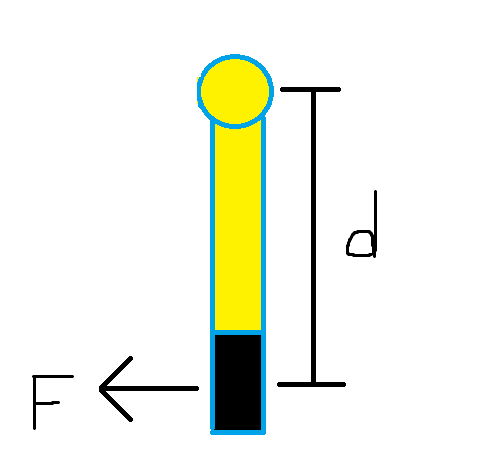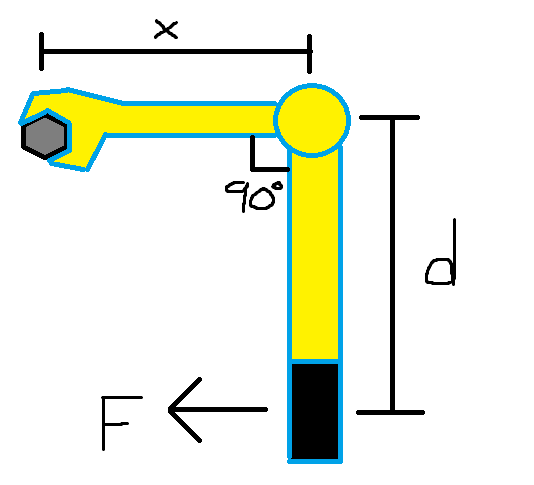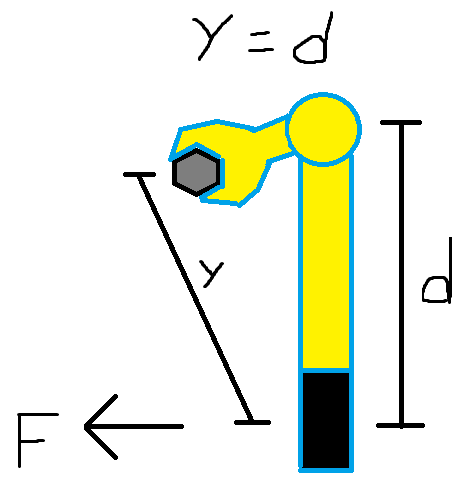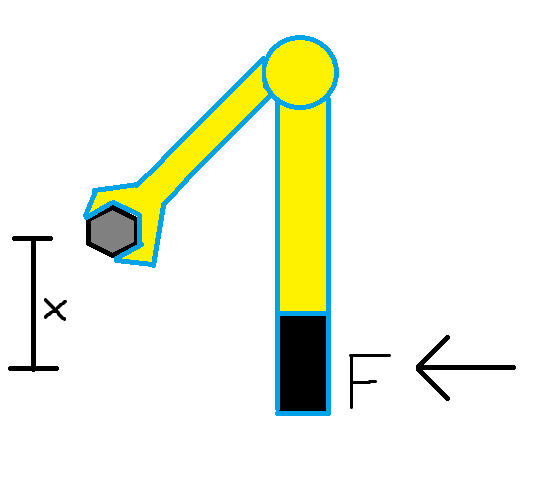# How is torque actually measured when using a torque wrench?Take this picture for example. Assume this picture represents a torque wrench applying torque on a nut, and a force F is applied at the centre of the black handle perpendicular to it. The force applied to the nut is:

Torque = Fd

There are times when a nut is in a very inconvenient location and you can't fit a torque wrench on it. Sometimes, a crow foot extension will be able to reach it. The problem is, changing the lever arm changes the torque.

Case 1: The common knowledge is that if the extension is at 90 degrees to the torque wrench, the torque applied to the nut doesn't change.

Torque = Fd (regardless of x)Case 2: I have also heard that in order for the torque to remain the same, the distance from the handle of the wrench to the nut should be kept the same as the distance from the handle to the pivot point.

Torque = Fy = FdCase 3: Distance for torque should be measured from the handle to the nut perpendicular to the force applied.

Torque = FxWhat is the correct method to measure torque applied to the nut?